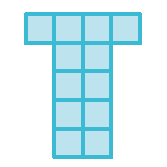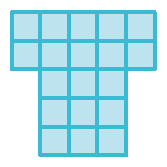### Home > CCAA8 > Chapter 2 Unit 2 > Lesson CCA: 2.1.1 > Problem2-6

2-6.

A tile pattern has 5 tiles in Figure 0 and adds 7 tiles in each new figure. Write the equation of the line that represents the growth of this pattern. 2-6 HW eTool (Desmos). Homework Help ✎.

Illustrate the problem.Figure 0 Figure 1 Figure 2

Use the equation of a line to substitute in known information.

The equation of a line is $y=mx+b$.

$m=$ the rate of growth between each figure
$b=$ the starting number of tiles

$y=7x+5$